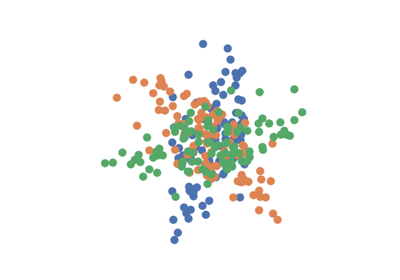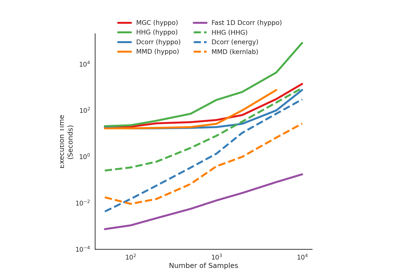# KSampleTest¶

class hyppo.ksample.base.KSampleTest(compute_distance=None, bias=False, **kwargs)

A base class for a k-sample test.

Parameters
• compute_distance (str, callable, or None, default: "euclidean" or "gaussian") -- A function that computes the distance among the samples within each data matrix. Valid strings for compute_distance are, as defined in sklearn.metrics.pairwise_distances,

• From scikit-learn: ["euclidean", "cityblock", "cosine", "l1", "l2", "manhattan"] See the documentation for scipy.spatial.distance for details on these metrics.

• From scipy.spatial.distance: ["braycurtis", "canberra", "chebyshev", "correlation", "dice", "hamming", "jaccard", "kulsinski", "mahalanobis", "minkowski", "rogerstanimoto", "russellrao", "seuclidean", "sokalmichener", "sokalsneath", "sqeuclidean", "yule"] See the documentation for scipy.spatial.distance for details on these metrics.

Alternatively, this function computes the kernel similarity among the samples within each data matrix. Valid strings for compute_kernel are, as defined in sklearn.metrics.pairwise.pairwise_kernels,

["additive_chi2", "chi2", "linear", "poly", "polynomial", "rbf", "laplacian", "sigmoid", "cosine"]

Note "rbf" and "gaussian" are the same metric.

• bias (bool (default: False)) -- Whether or not to use the biased or unbiased test statistics. Only applies to Dcorr and Hsic.

• **kwargs -- Arbitrary keyword arguments for compute_distkern.

Methods Summary

 Calulates the k-sample test statistic. KSampleTest.test(*args[, reps, workers]) Calculates the k-sample test statistic and p-value.

abstract KSampleTest.statistic(*args)

Calulates the k-sample test statistic.

Parameters

*args (ndarray) -- Variable length input data matrices. All inputs must have the same number of dimensions. That is, the shapes must be (n, p) and (m, p), ... where n, m, ... are the number of samples and p is the number of dimensions.

Returns

stat (float) -- The computed k-Sample statistic.

abstract KSampleTest.test(*args, reps=1000, workers=1)

Calculates the k-sample test statistic and p-value.

Parameters
• *args (ndarray) -- Variable length input data matrices. All inputs must have the same number of dimensions. That is, the shapes must be (n, p) and (m, p), ... where n, m, ... are the number of samples and p is the number of dimensions.

• reps (int, default: 1000) -- The number of replications used to estimate the null distribution when using the permutation test used to calculate the p-value.

• workers (int, default: 1) -- The number of cores to parallelize the p-value computation over. Supply -1 to use all cores available to the Process.

Returns

## Examples using hyppo.ksample.base.KSampleTest¶